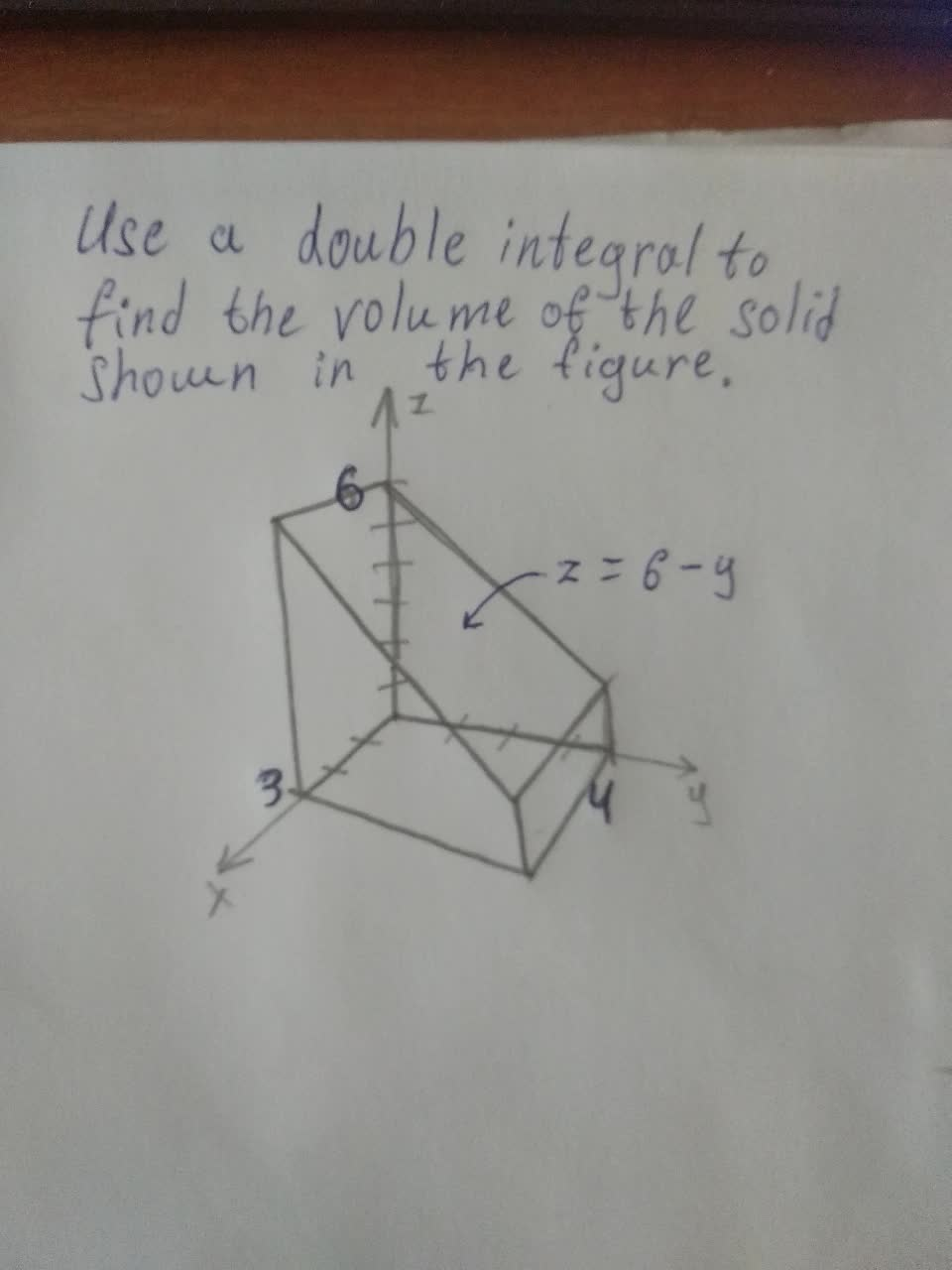# Calculus 1: integral problems solved# Recent questions in Integrals

Integrals
ANSWERED### Evaluate the line integral, where C is the given curve. $$\int y3\ ds,\ C\div x=t3,\ y=t,\ 0?\ t?\ 3$$

Integrals
ANSWERED### Evaluate the line integral, where C is the given curve. C $$y^3 ds, C: x = t^3, y = t, 0 \leq t \leq 4$$

Integrals
ANSWERED### Evaluate the integral $$\int \arctan(x)dx$$ A)$$-\arctan(x)+\ln(x^2+1)+C$$ B)$$\frac{1}{x^2+1}+C$$ C)$$x \arctan(x)-\frac{1}{2}\ln(x^2+1)+C$$ D) $$\ln|\arccos(x)|+C$$ E) $$x\arctan(x)+C$$

Integrals
ANSWERED### Evaluate the line integral $$\int_c xyds$$ Where c is the given curve $$c:x=t^2,y=2t,0\leq t\leq5$$

Integrals
ANSWERED### Use a double integral to find the area of the region. The region inside the circle $$(x - 5)^2 + y^2 = 25$$ and outside the circle $$x^2 + y^2 = 25$$

Integrals
ANSWERED### Evaluate the indefinite integral as an infinite series. $$\int \frac{\cos x-1}{x} dx$$

Integrals
ANSWERED### Find the exact length of the curve. $$x = \frac{y^4}{8} + \frac{1}{4y^2} , 1 \leq y \leq 2$$

Integrals
ANSWERED### Use a double intergral to find a volume of the solid shown in the figureIntegrals
ANSWERED### Evaluate the indefinite integral as a power series. $$\int \frac{\tan^{-1}x}{x}dx$$ $$f(x)=C+\sum_{n=0}^\infty\left( \dots \right)$$ What is the radius of convergence R?

Integrals
ANSWERED### Evaluate the surface integral. $$\int \int_s (x^2z +y^2z)dS$$ S is the hemisphere $$x^2 +y^2 + z^2 = 9, z \geq 0$$

Integrals
ANSWERED### Find the area of the part of the plane $$5x + 4y + z = 20$$ that lies in the first octant.

Integrals
ANSWERED### Evaluate the line integral, where C is the given curve.C $$y^3 ds, C: x = t^3, y = t, 0 \leq t \leq 5$$

Integrals
ANSWERED### Determine whether the following integrals are convergen: $$\int_5^\infty\frac{\arctan x}{x^2+3x+5}dx$$

Integrals
ANSWERED### Use the Table of Integrals to evaluate the integral. (Use C for the constant of integration.) $$7\sin^{8}(x)\cos(x)\ln(\sin(x))dx$$ no. 101. $$\displaystyle\int{u}^{{{n}}} \ln{{u}}{d}{u}={\frac{{{u}^{{{n}+{1}}}}}{{{\left({n}+{1}\right)}^{{{2}}}}}}{\left[{\left({n}+{1}\right)} \ln{{u}}-{1}\right]}+{C}$$

Integrals
ANSWERED### Find the exact length of the curve. $$y=\sqrt{x-x^2}+\sin ^{-1}\sqrt{x}$$

Integrals
ANSWERED### Find the area of the part of the plane $$5x + 3y + z = 15$$ that lies in the first octant.

Integrals
ANSWERED### a) Write the sigma notation formula for the right Riemann sum $$R_{n}$$ of the function $$f(x)=4-x^{2}$$ on the interval $$[0,\ 2]$$ using n subintervals of equal length, and calculate the definite integral $$\int_{0}^{2}f(x) dx$$ as the limit of $$R_{n}$$ at $$n\rightarrow\infty$$. (Reminder: $$\sum_{k=1}^{n}k=\frac{n(n+1)}{2},\ \sum_{k=1}^{n}k^{2}=\frac{n(n+1)(2n+1)}{6}$$ b) Use the Fundamental Theorem of Calculus to calculate the derivative of $$F(x)=\int_{e^{-x}}^{x}\ln(t^{2}+1)dt$$

Integrals
ANSWERED### Use the Table of Integrals to evaluate the integral. (Use C for the constant of integration.) $$\int37e^{74x}\arctan(e^{37x})dx$$ Inverse Trigonometric Forms (92): $$\int u\tan^{-1}u\ du=\frac{u^{2}+1}{2}\tan^{-1}u-\frac{u}{2}+C$$

Integrals
ANSWERED### Calculate the double integral. $$\int \int_R \frac{4x}{1+xy}dA$$ $$R=\left[0,4\right] \times \left[0,1\right]$$
ANSWERED